# Euler angles

The Euler angles are three angles introduced by Leonhard Euler to describe the orientation of a rigid body with respect to a fixed coordinate system. They can also represent the orientation of a mobile frame of reference in physics or the orientation of a general basis in 3-dimensional linear algebra.

Any orientation can be achieved by composing three elemental rotations, i.e. rotations about the axes of a coordinate system. Euler angles can be defined by three of these rotations. They can also be defined by elemental geometry and the geometrical definition demonstrates that three rotations are always sufficient to reach any frame.

The three elemental rotations may be extrinsic (rotations about the axes xyz of the original coordinate system, which is assumed to remain motionless), or intrinsic (rotations about the axes of the rotating coordinate system XYZ, solidary with the moving body, which changes its orientation after each elemental rotation).

Euler angles are typically denoted as α, β, γ, or φ, θ, ψ. Different authors may use different sets of rotation axes to define Euler angles, or different names for the same angles. Therefore, any discussion employing Euler angles should always be preceded by their definition.

Without considering the possibility of using two different conventions for the definition of the rotation axes (intrinsic or extrinsic), there exist twelve possible sequences of rotation axes, divided in two groups:

• Proper Euler angles (z-x-z, x-y-x, y-z-y, z-y-z, x-z-x, y-x-y)
• Tait–Bryan angles (x-y-z, y-z-x, z-x-y, x-z-y, z-y-x, y-x-z).

Tait–Bryan angles are also called Cardan angles; nautical angles; heading, elevation, and bank; or yaw, pitch, and roll. Sometimes, both kinds of sequences are called "Euler angles". In that case, the sequences of the first group are called proper or classic Euler angles.

## Proper Euler angles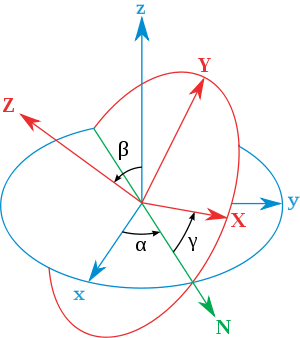Proper Euler angles geometrical definition. The xyz (fixed) system is shown in blue, the XYZ (rotated) system is shown in red. The line of nodes (N) is shown in green

### Geometrical definition

The axes of the original frame are denoted as x,y,z and the axes of the rotated frame are denoted as X,Y,Z. The geometrical definition (sometimes referred to as static) begins defining the line of nodes as the intersection of the planes xy and the XY (it can also be defined as the common perpendicular to the axes z and Z and then written as the vector product N = Z x z). Using it, the three Euler angles can be defined as follows:

• α (or) is the angle between the x axis and the N axis (x-convention. It could also be defined between y and N, called y-convention).
• β (or) is the angle between the z axis and the Z axis.
• γ (or) is the angle between the N axis and the X axis (x-convention).

Euler angles between two reference frames are defined only if both frames have the same handedness.

### Definition by intrinsic rotations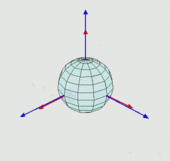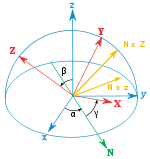Any target orientation can be reached, starting from a known reference orientation, using a specific sequence of intrinsic rotations, whose magnitudes are the Euler angles of the target orientation. This example uses the z-x′-z″ sequence.

Intrinsic rotations are elemental rotations that occur about the axes of a coordinate system XYZ attached to a moving body. Therefore they change their orientation after each elemental rotation. The XYZ system rotates, while xyz is fixed. Starting with XYZ overlapping xyz, a composition of three intrinsic rotations can be used to reach any target orientation for XYZ.

Euler angles can be defined by intrinsic rotations. The rotated frame XYZ may be imagined to be initially aligned with xyz, before undergoing the three elemental rotations represented by Euler angles. Its successive orientations may be denoted as follows:

• x-y-z, or x0-y0-z0 (initial)
• x’-y’-z’, or x1-y1-z1 (after first rotation)
• x″-y″-z″, or x2-y2-z2 (after second rotation)
• X-Y-Z, or x3-y3-z3 (final)

For the above-listed sequence of rotations, the line of nodes N can be simply defined as the orientation of X after the first elemental rotation. Hence, N can be simply denoted x’. Moreover, since the third elemental rotation occurs about Z, it does not change the orientation of Z. Hence Z coincides with z″. This allows us to simplify the definition of the Euler angles as follows:

• α (or) represents a rotation around the z axis,
• β (or) represents a rotation around the x’ axis,
• γ (or) represents a rotation around the z″ axis.

### Definition by extrinsic rotations

Extrinsic rotations are elemental rotations that occur about the axes of the fixed coordinate system xyz. The XYZ system rotates, while xyz is fixed. Starting with XYZ overlapping xyz, a composition of three extrinsic rotations can be used to reach any target orientation for XYZ. The Euler or Tait-Bryan angles (α, β, γ) are the amplitudes of these elemental rotations. For instance, the target orientation can be reached as follows:

• The XYZ system rotates about the z axis by α. The X axis is now at angle α with respect to the x axis.
• The XYZ system rotates again about the x axis by β. The Z axis is now at angle β with respect to the z axis.
• The XYZ system rotates a third time about the z axis by γ.

In sum, the three elemental rotations occur about z, x and z. Indeed, this sequence is often denoted z-x-z (or 3-1-3). Sets of rotation axes associated with both proper Euler angles and Tait–Bryan angles are commonly named using this notation (see above for details).

### Conventions

There are six possibilities of choosing the rotation axes for proper Euler angles. In all of them, the first and third rotation axes are the same. The six possible sequences are:

1. z-x’-z″ (intrinsic rotations) or z-x-z (extrinsic rotations)
2. x-y’-x″ (intrinsic rotations) or x-y-x (extrinsic rotations)
3. y-z’-y″ (intrinsic rotations) or y-z-y (extrinsic rotations)
4. z-y’-z″ (intrinsic rotations) or z-y-z (extrinsic rotations)
5. x-z’-x″ (intrinsic rotations) or x-z-x (extrinsic rotations)
6. y-x’-y″ (intrinsic rotations) or y-x-y (extrinsic rotations)

### Signs and ranges

Angles are commonly defined according to the right hand rule. Namely, they have positive values when they represent a rotation that appears clockwise when looking in the positive direction of the axis, and negative values when the rotation appears counter-clockwise. The opposite convention (left hand rule) is less frequently adopted.

About the ranges (using interval notation):

• for α and γ, the range is defined moduloradians. For instance, a valid range could be [π, π).
• for β, the range covers π radians (but can't be said to be modulo π). For example, it could be [0, π] or [π/2, π/2].

The angles α, β and γ are uniquely determined except for the singular case that the xy and the XY planes are identical, i.e. when the z axis and the Z axis have the same or opposite directions. Indeed, if the z axis and the Z axis are the same, β = 0 and only (α + γ) is uniquely defined (not the individual values), and, similarly, if the z axis and the Z axis are opposite, β = π and only (α  γ) is uniquely defined (not the individual values). These ambiguities are known as gimbal lock in applications.

### Precession, nutation and intrinsic rotation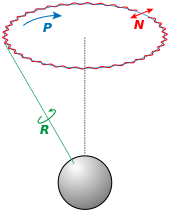Euler basic motions of the Earth. Intrinsic (green), Precession (blue) and Nutation (red)

Precession, nutation, and intrinsic rotation (spin) are defined as the movements obtained by changing one of the Euler angles while leaving the other two constant. These motions are not expressed in terms of the external frame, or in terms of the co-moving rotated body frame, but in a mixture. They constitute a mixed axes of rotation system, where the first angle moves the line of nodes around the external axis z, the second rotates around the line of nodes and the third one is an intrinsic rotation around an axis fixed in the body that moves.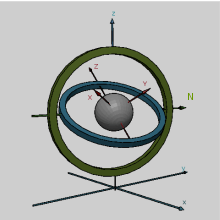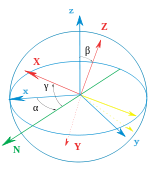Left: A gimbal set, showing a z-x-z rotation sequence. External frame shown in the base. Internal axes in red color. Right: A simple diagram showing similar Euler angles in a diagram.

The static definition implies that:

• α represents a rotation around the z axis,
• β represents a rotation around the N axis,
• γ represents a rotation around the Z axis.

If β is zero, there is no rotation about N. As a consequence, Z coincides with z, α and γ represent rotations about the same axis (z), and the final orientation can be obtained with a single rotation about z, by an angle equal to α+γ.

As an example, consider a top. The top spins around its own axis of symmetry; this corresponds to its intrinsic rotation. It also rotates around its pivotal axis, with its center of mass orbiting the pivotal axis; this rotation is a precession. Finally, the top can wobble up and down; the inclination angle is the nutation angle. The same example can be seen with the movements of the earth.

Though all three movements can be represented by a rotation operator with constant coefficients in some frame, they cannot be represented by these operators all at the same time. Given a reference frame, at most one of them will be coefficient-free. Only precession can be expressed in general as a matrix in the basis of the space without dependencies of the other angles.

These movements also behave as a gimbal set. If we suppose a set of frames, able to move each with respect to the former according to just one angle, like a gimbal, there will exist an external fixed frame, one final frame and two frames in the middle, which are called "intermediate frames". The two in the middle work as two gimbal rings that allow the last frame to reach any orientation in space.

## Tait–Bryan angles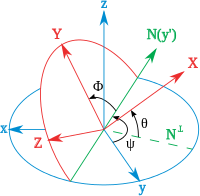Tait–Bryan angles. z-y′-x″ sequence (intrinsic rotations; N coincides with y’). The angle rotation sequence is ψ, θ, Ф. Note that in this case ψ > 90° and θ is a negative angle.

The second type of formalism is called Tait–Bryan angles, after Peter Guthrie Tait and George H. Bryan. It is the convention normally used for aerospace applications, so that zero degrees elevation represents the horizontal attitude. Tait–Bryan angles represent the orientation of the aircraft respect the world frame. When dealing with other vehicles, different axes conventions are possible.

### Definitions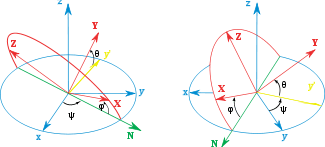Tait–Bryan angles. z-x’-y″ sequence (intrinsic rotations; N coincides with x’)

The definitions and notations used for Tait-Bryan angles are similar to those described above for proper Euler angles (geometrical definition, intrinsic rotation definition, extrinsic rotation definition). The only difference is that Tait–Bryan angles represent rotations about three distinct axes (e.g. x-y-z, or x-y’-z″), while proper Euler angles use the same axis for both the first and third elemental rotations (e.g., z-x-z, or z-x’-z″).

This implies a different definition for the line of nodes in the geometrical construction. In the proper Euler angles case it was defined as the intersection between two homologous Cartesian planes (parallel when Euler angles are zero; e.g. xy and XY). In the second one, it is defined as the intersection of two non-homologous planes (perpendicular when Euler angles are zero; e.g. xy and YZ).

### Conventions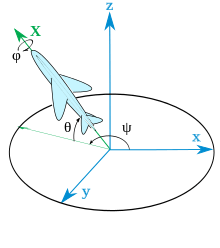Heading, elevation and bank angles (Z-Y’-X’’) for an aircraft using onboard ENU axes both onboard and for the ground tracking station. The fixed reference frame x-y-z represents such a tracking station. Onboard axes Y and Z are not shown. X shown in green color.

The three elemental rotations may occur either about the axes of the original coordinate system, which remains motionless (extrinsic rotations), or about the axes of the rotating coordinate system, which changes its orientation after each elemental rotation (intrinsic rotations).

There are six possibilities of choosing the rotation axes for Tait–Bryan angles. The six possible sequences are:

• x-y’-z″ (intrinsic rotations) or x-y-z (extrinsic rotations)
• y-z’-x″ (intrinsic rotations) or y-z-x (extrinsic rotations)
• z-x’-y″ (intrinsic rotations) or z-x-y (extrinsic rotations)
• x-z’-y″ (intrinsic rotations) or x-z-y (extrinsic rotations)
• z-y’-x″ (intrinsic rotations) or z-y-x (extrinsic rotations): the intrinsic rotations are known as: yaw, pitch and roll
• y-x’-z″ (intrinsic rotations) or y-x-z (extrinsic rotations)

### Signs and ranges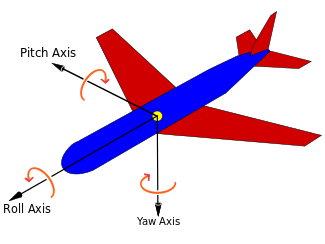The principal axes of an aircraft according to the air norm DIN 9300. Notice that fixed and mobile frames must be coincident with angles zero. Therefore this norm would force also a compatible axes convention in the reference system

Tait-Bryan convention is widely used in engineering with different purposes. There are several axes conventions in practice for choosing the mobile and fixed axes, and these conventions determine the signs of the angles. Therefore signs must be studied in each case carefully.

The range for the angles ψ and φ covers 2π radians. For θ the range covers π.

### Alternative names

These angles are normally taken as one in the external reference frame (heading, bearing), one in the intrinsic moving frame (bank) and one in a middle frame, representing an elevation or inclination respect the horizontal plane, which is equivalent to the line of nodes for this purpose.

For an aircraft, they can be obtained with three rotations around its principal axes if done in the proper order. A yaw will obtain the bearing, a pitch will yield the elevation and a roll gives the bank angle. Therefore in aerospace they are sometimes called yaw, pitch and roll. Notice that this will not work if the rotations are applied in any other order or if the airplane axes start in any position non-equivalent to the reference frame.

Tait-Bryan angles, following z-y’-x″ (intrinsic rotations) convention, are also known as nautical angles, because they can be used to describe the orientation of a ship or aircraft, or Cardan angles, after the Italian mathematician and physicist Gerolamo Cardano, who first described in detail the Cardan suspension and the Cardan joint.

## Angles of a given frame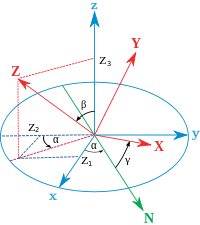Projections of Z vector.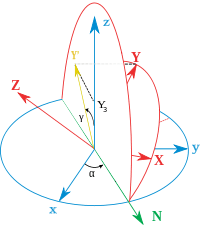Projections of Y vector.

A common problem is to find the Euler angles of a given frame. The fastest way to get them is to write the three given vectors as columns of a matrix and compare it with the expression of the theoretical matrix (see later table of matrices). Hence the three Euler Angles can be calculated. Nevertheless, the same result can be reached avoiding matrix algebra and using only elemental geometry. Here we present the results for the two most commonly used conventions: ZXZ for proper Euler angles and ZYX for Tait-Bryan. Notice that any other convention can be obtained just changing the name of the axes.

### Proper Euler angles

Assuming a frame with unit vectors (X, Y, Z) given by their coordinates as in the main diagram, it can be seen that:And, sincewe haveAsis the double projection of a unitary vector,There is a similar construction for, projecting it first over the plane defined by the axis z and the line of nodes. As the angle between the planes isand, this leads to:and finally, using the inverse cosine function,### Tait-Bryan angles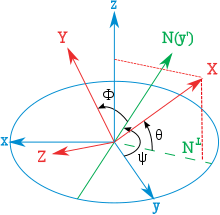projections of X axis after three Tait-Bryan rotations. Notice that theta is a negative rotation around the axis y'

Assuming a frame with unit vectors (X, Y, Z) given by their coordinates as in this new diagram (notice that the angle theta is negative), it can be seen that:As before,we havein a way analogous to the former one:Looking for similar expressions to the former ones:### Last remarks

It is interesting to note that the inverse sine and cosine functions yield two possible values for the argument. In this geometrical description only one of the solutions is valid. When Euler Angles are defined as a sequence of rotations, all the solutions can be valid, but there will be only one inside the angle ranges. This is because the sequence of rotations to reach the target frame is not unique if the ranges are not previously defined.

For computational purposes, it may be useful to represent the angles using atan2(y,x). For example in the case of proper Euler angles:## Conversion to other orientation representations

Euler angles are one way to represent orientations. There are others, and it is possible to change to and from other conventions. Three parameters are always required to describe orientations in a 3-dimensional Euclidean space. They can be given in several ways, Euler angles being one of them; see charts on SO(3) for others.

The most used orientation representation are the rotation matrices, the axis-angle and the quaternions, also known as Euler–Rodrigues parameters, which provide another mechanism for representing 3D rotations. This is equivalent to the special unitary group description.

Expressing rotations in 3D as unit quaternions instead of matrices has some advantages:

• Concatenating rotations is computationally faster and numerically more stable.
• Extracting the angle and axis of rotation is simpler.
• Interpolation is more straightforward. See for example slerp.
• Quaternions do not suffer from gimbal lock as Euler angles do.

Anyway, the rotation matrix calculation is the first step for obtaining the other two representations.

### Rotation matrix

Any orientation can be achieved by composing three elemental rotations, starting from a known standard orientation. Equivalently, any rotation matrix R can be decomposed as a product of three elemental rotation matrices. For instance:is a rotation matrix that may be used to represent a composition of intrinsic rotations about axes x-y’-z″. However, both the definition of the elemental rotation matrices X, Y, Z, and their multiplication order depend on the choices taken by the user about the definition of both rotation matrices and Euler angles (see, for instance, Ambiguities in the definition of rotation matrices). Unfortunately, different sets of conventions are adopted by users in different contexts. The following table was built according to this set of conventions:

1. Each matrix is meant to operate by pre-multiplying column vectors (see Ambiguities in the definition of rotation matrices)
2. Each matrix is meant to represent an active rotation (the composing and composed matrices are supposed to act on the coordinates of vectors defined in the initial fixed reference frame and give as a result the coordinates of a rotated vector defined in the same reference frame).
3. Each matrix is meant to represent the composition of intrinsic rotations (around the axes of the rotating reference frame).
4. Right handed reference frames are adopted, and the right hand rule is used to determine the sign of the angles α, β, γ.

For the sake of simplicity, the following table uses the following nomenclature:

1. 1, 2, 3 represent the angles α, β, γ.
2. X, Y, Z are the matrices representing the elemental rotations about the axes x, y, z of the fixed frame (e.g., X1 represents a rotation about x by an angle α).
3. s and c represent sine and cosine (e.g., s1 represents the sine of α).
4. Each matrix is denoted by the formula used to calculate it. If, we name it.
Proper Euler angles Tait-Bryan anglesTo change the formulas for the opposite direction of rotation, change the signs of the sine functions. To change the formulas for passive rotations, transpose the matrices (then each matrix transforms the initial coordinates of a vector remaining fixed to the coordinates of the same vector measured in the rotated reference system; same rotation axis, same angles, but now the coordinate system rotates, rather than the vector).

## Properties

The Euler angles form a chart on all of SO(3), the special orthogonal group of rotations in 3D space. The chart is smooth except for a polar coordinate style singularity along β=0. See charts on SO(3) for a more complete treatment.

The space of rotations is called in general "The Hypersphere of rotations", though this is a misnomer: the group Spin(3) is isometric to the hypersphere S3, but the rotation space SO(3) is instead isometric to the real projective space RP3 which is a 2-fold quotient space of the hypersphere. This 2-to-1 ambiguity is the mathematical origin of spin in physics.

A similar three angle decomposition applies to SU(2), the special unitary group of rotations in complex 2D space, with the difference that β ranges from 0 to 2π. These are also called Euler angles.

The Haar measure for Euler angles has the simple form sin(β)..., usually normalized by a factor of 1/8π².

For example, to generate uniformly randomized orientations, let α and γ be uniform from 0 to 2π, let z be uniform from 1 to 1, and let β = arccos(z).

### Geometric algebra

Other properties of Euler angles and rotations in general can be found from the Geometric algebra(GA). GA is a higher level abstraction, in which the quaternions are an even subalgebra. The principal tool in GA is the rotorwhereangle of rotation,rotation axis (unitary vector) andpseudoscalar (trivector in)

### Higher dimensions

It is possible to define parameters analogous to the Euler angles in dimensions higher than three.

The number of degrees of freedom of a rotation matrix is always less than the dimension of the matrix squared. That is, the elements of a rotation matrix are not all completely independent. For example, the rotation matrix in dimension 2 has only one degree of freedom, since all four of its elements depend on a single angle of rotation. A rotation matrix in dimension 3 (which has nine elements) has three degrees of freedom, corresponding to each independent rotation, for example by its three Euler angles or a magnitude one (unit) quaternion.

In SO(4) the rotation matrix is defined by two quaternions, and is therefore 6-parametric (three degrees of freedom for every quaternion). The 4×4 rotation matrices have therefore 6 out of 16 independent components.

Any set of 6 parameters that define the rotation matrix could be considered an extension of Euler angles to dimension 4.

In general, the number of euler angles in dimension D is quadratic in D; since any one rotation consists of choosing two dimensions to rotate between, the total number of rotations available in dimensionis, which foryields.

## Applications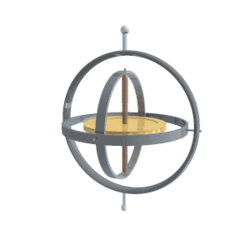A gyroscope keeps its rotation axis constant. Therefore, angles measured in this frame are equivalent to angles measured in the lab frame

### Vehicles and moving frames

Main article: rigid body

Their main advantage over other orientation descriptions is that they are directly measurable from a gimbal mounted in a vehicle. As gyroscopes keep their rotation axis constant, angles measured in a gyro frame are equivalent to angles measured in the lab frame. Therefore, gyros are used to know the actual orientation of moving spacecraft, and Euler angles are directly measurable. Intrinsic rotation angle cannot be read from a single gimbal, so there has to be more than one gimbal in a spacecraft. Normally there are at least three for redundancy. There is also a relation to the well-known gimbal lock problem of mechanical engineering  .

When studying rigid bodies in general, one calls the xyz system space coordinates, and the XYZ system body coordinates. The space coordinates are treated as unmoving, while the body coordinates are considered embedded in the moving body. Calculations involving acceleration, angular acceleration, angular velocity, angular momentum, and kinetic energy are often easiest in body coordinates, because then the moment of inertia tensor does not change in time. If one also diagonalizes the rigid body's moment of inertia tensor (with nine components, six of which are independent), then one has a set of coordinates (called the principal axes) in which the moment of inertia tensor has only three components.

The angular velocity of a rigid body takes a simple form using Euler angles in the moving frame. Also the Euler's rigid body equations are simpler because the inertia tensor is constant in that frame.

### Crystallographic texture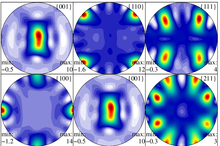Pole figures displaying crystallographic texture of gamma-TiAl in an alpha2-gamma alloy, as measured by high energy X-rays.

In materials science, crystallographic texture (or preferred orientation) can be described using Euler angles. In texture analysis, the Euler angles provide a mathematical depiction of the orientation of individual crystallites within a polycrystalline material, allowing for the quantitative description of the macroscopic material. The most common definition of the angles is due to Bunge and corresponds to the ZXZ convention. It is important to note, however, that the application generally involves axis transformations of tensor quantities, i.e. passive rotations. Thus the matrix that corresponds to the Bunge Euler angles is the transpose of that shown in the table above.

### OthersIndustrial robot operating in a foundry.

Euler angles, normally in the Tait–Bryan convention, are also used in robotics for speaking about the degrees of freedom of a wrist. They are also used in Electronic stability control in a similar way.

Gun fire control systems require corrections to gun-order angles (bearing and elevation) to compensate for deck tilt (pitch and roll). In traditional systems, a stabilizing gyroscope with a vertical spin axis corrects for deck tilt, and stabilizes the optical sights and radar antenna. However, gun barrels point in a direction different from the line of sight to the target, to anticipate target movement and fall of the projectile due to gravity, among other factors. Gun mounts roll and pitch with the deck plane, but also require stabilization. Gun orders include angles computed from the vertical gyro data, and those computations involve Euler angles.

Euler angles are also used extensively in the quantum mechanics of angular momentum. In quantum mechanics, explicit descriptions of the representations of SO(3) are very important for calculations, and almost all the work has been done using Euler angles. In the early history of quantum mechanics, when physicists and chemists had a sharply negative reaction towards abstract group theoretic methods (called the Gruppenpest), reliance on Euler angles was also essential for basic theoretical work.

Many mobile computing devices contain accelerometers which can determine these devices' Euler angles with respect to the earth's gravitational attraction. These are used in applications such as games, bubble level simulations, and kaleidoscopes.

1. Novi Commentarii academiae scientiarum Petropolitanae 20, 1776, pp. 189–207 (E478) pdf
2. Gregory G. Slabaugh, Computing Euler angles from a rotation matrix
3. The relation between the Euler angles and the Cardan suspension is explained in chap. 11.7 of the following textbook: U. Krey, A. Owen, Basic Theoretical Physics – A Concise Overview, New York, London, Berlin, Heidelberg, Springer (2007) .
4. Liss KD, Bartels A, Schreyer A, Clemens H (2003). "High energy X-rays: A tool for advanced bulk investigations in materials science and physics". Textures Microstruct. 35 (3/4): 219–52. doi:10.1080/07303300310001634952.
5. Kocks, U.F.; Tomé, C.N.; Wenk, H.-R. (2000), Texture and Anisotropy: Preferred Orientations in Polycrystals and their effect on Materials Properties, Cambridge, ISBN 978-0-521-79420-6
6. Bunge, H. (1993), Texture Analysis in Materials Science: Mathematical Methods, CUVILLIER VERLAG, ASIN B0014XV9HU

## Bibliography

• Biedenharn, L. C.; Louck, J. D. (1981), Angular Momentum in Quantum Physics, Reading, MA: Addison–Wesley, ISBN 978-0-201-13507-7
• Goldstein, Herbert (1980), Classical Mechanics (2nd ed.), Reading, MA: Addison–Wesley, ISBN 978-0-201-02918-5
• Gray, Andrew (1918), A Treatise on Gyrostatics and Rotational Motion, London: Macmillan (published 2007), ISBN 978-1-4212-5592-7
• Rose, M. E. (1957), Elementary Theory of Angular Momentum, New York, NY: John Wiley & Sons (published 1995), ISBN 978-0-486-68480-2Wikimedia Commons has media related to Euler angles.